xCronbach's alphaEncyclopedia
Cronbach'sis a coefficient of reliability
Reliability (statistics)
In statistics, reliability is the consistency of a set of measurements or of a measuring instrument, often used to describe a test. Reliability is inversely related to random error.-Types:There are several general classes of reliability estimates:...

. It is commonly used as a measure of the internal consistency
Internal consistency
In statistics and research, internal consistency is typically a measure based on the correlations between different items on the same test . It measures whether several items that propose to measure the same general construct produce similar scores...

or reliability
Reliability (statistics)
In statistics, reliability is the consistency of a set of measurements or of a measuring instrument, often used to describe a test. Reliability is inversely related to random error.-Types:There are several general classes of reliability estimates:...

of a psychometric test score for a sample of examinees. It was first named alpha by Lee Cronbach
Lee Cronbach
Lee Joseph Cronbach was an American educational psychologist who made significant contributions to psychological testing and measurement. Born in Fresno, California, Cronbach was selected as a child to participate in Lewis Terman's long-term study of talented children...

in 1951, as he had intended to continue with further coefficients. The measure can be viewed as an extension of the Kuder-Richardson Formula 20
Kuder-Richardson Formula 20
In statistics, the Kuder-Richardson Formula 20 first published in 1937 is a measure of internal consistency reliability for measures with dichotomous choices. It is analogous to Cronbach's α, except Cronbach's α is also used for non-dichotomous measures...

(KR-20), which is an equivalent measure for dichotomous
Dichotomy
A dichotomy is any splitting of a whole into exactly two non-overlapping parts, meaning it is a procedure in which a whole is divided into two parts...

items. Alpha is not robust
Robust statistics
Robust statistics provides an alternative approach to classical statistical methods. The motivation is to produce estimators that are not unduly affected by small departures from model assumptions.- Introduction :...

against missing data. Several other Greek letters have been used by later researchers to designate other measures used in a similar context. Somewhat related is the average variance extracted (AVE).

This article discusses the use ofin psychology, but Cronbach's alpha statistic is widely used in the social sciences
Social sciences
Social science is the field of study concerned with society. "Social science" is commonly used as an umbrella term to refer to a plurality of fields outside of the natural sciences usually exclusive of the administrative or managerial sciences...

, business, nursing, and other disciplines. The term item is used throughout this article, but items could be anything — questions, raters, indicators — of which one might ask to what extent they "measure the same thing." Items that are manipulated are commonly referred to as variables.

## Definition

Cronbach'sis defined as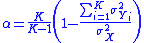where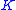is the number of components (K-items or testlets),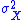the variance
Variance
In probability theory and statistics, the variance is a measure of how far a set of numbers is spread out. It is one of several descriptors of a probability distribution, describing how far the numbers lie from the mean . In particular, the variance is one of the moments of a distribution...

of the observed total test scores, and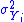the variance of component i for the current sample of persons. See Develles (1991).

Alternatively, the Cronbach'scan also be defined as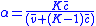whereis as above,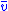the average variance, and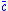the average of all covariance
Covariance
In probability theory and statistics, covariance is a measure of how much two variables change together. Variance is a special case of the covariance when the two variables are identical.- Definition :...

s between the components across the current sample of persons.

The standardized Cronbach's alpha can be defined as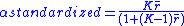whereis as above and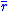the mean of the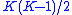non-redundant correlation coefficients (i.e., the mean of an upper triangular
Triangular matrix
In the mathematical discipline of linear algebra, a triangular matrix is a special kind of square matrix where either all the entries below or all the entries above the main diagonal are zero...

, or lower triangular, correlation matrix).

Cronbach'sis related conceptually to the Spearman–Brown prediction formula. Both arise from the basic classical test theory
Classical test theory
Classical test theory is a body of related psychometric theory that predict outcomes of psychological testing such as the difficulty of items or the ability of test-takers. Generally speaking, the aim of classical test theory is to understand and improve the reliability of psychological...

result that the reliability of test scores can be expressed as the ratio of the true-score and total-score (error plus true score) variances: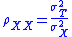Theoretically, alpha varies from zero to 1, since it is the ratio of two variances. Empirically, however, alpha can take on any value less than or equal to 1, including negative values, although only positive values make sense. Higher values of alpha are more desirable. Some professionals as a rule of thumb
Rule of thumb
A rule of thumb is a principle with broad application that is not intended to be strictly accurate or reliable for every situation. It is an easily learned and easily applied procedure for approximately calculating or recalling some value, or for making some determination...

, require a reliability of 0.70 or higher (obtained on a substantial sample) before they will use an instrument. Obviously, this rule should be applied with caution whenhas been computed from items that systematically violate its assumptions. Furthermore, the appropriate degree of reliability depends upon the use of the instrument. For example, an instrument designed to be used as part of a battery of tests may be intentionally designed to be as short as possible, and therefore somewhat less reliable. Other situations may require extremely precise measures with very high reliabilities.

This has resulted in a wide variance of test reliability. In the case of psychometric tests, most fall within the range of 0.75 to 0.83 with at least one claiming a Cronbach alpha above 0.90 (Nunnally 1978, page 245-246).

## Internal consistency

Cronbach's alpha will generally increase as the intercorrelations among test items increase, and is thus known as an internal consistency
Internal consistency
In statistics and research, internal consistency is typically a measure based on the correlations between different items on the same test . It measures whether several items that propose to measure the same general construct produce similar scores...

estimate of reliability of test scores. Because intercorrelations among test items are maximized when all items measure the same construct
Construct (philosophy of science)
A construct in the philosophy of science is an ideal object, where the existence of the thing may be said to depend upon a subject's mind. This, as opposed to a "real" object, where existence does not seem to depend on the existence of a mind....

, Cronbach's alpha is widely believed to indirectly indicate the degree to which a set of items measures a single unidimensional latent construct. However, the average intercorrelation among test items is affected by skew just like any other average. Thus, whereas the modal intercorrelation among test items will equal zero when the set of items measures several unrelated latent constructs, the average intercorrelation among test items will be greater than zero in this case. Indeed, several investigators have shown that alpha can take on quite high values even when the set of items measures several unrelated latent constructs (e.g., Cortina, 1993; Cronbach, 1951; Green, Lissitz & Mulaik, 1977; Revelle, 1979; Schmitt, 1996; Zinbarg, Yovel, Revelle & McDonald, 2006). As a result, alpha is most appropriately used when the items measure different substantive areas within a single construct. When the set of items measures more than one construct, coefficient omega_hierarchical is more appropriate (McDonald, 1999; Zinbarg, Revelle, Yovel & Li, 2005).

Alpha treats any covariance among items as true-score variance, even if items covary for spurious reasons. For example, alpha can be artificially inflated by making scales which consist of superficial changes to the wording within a set of items or by analyzing speeded tests.

A commonly accepted rule of thumb for describing internal consistency using Cronbach's alpha is as follows:
Cronbach's alpha
Cronbach's alpha
Cronbach's \alpha is a coefficient of reliability. It is commonly used as a measure of the internal consistency or reliability of a psychometric test score for a sample of examinees. It was first named alpha by Lee Cronbach in 1951, as he had intended to continue with further coefficients...

Internal consistency
α ≥ .9 Excellent
.9 > α ≥ .8 Good
.8 > α ≥ .7 Acceptable
.7 > α ≥ .6 Questionable
.6 > α ≥ .5 Poor
.5 > α Unacceptable

## Generalizability theory

Cronbach and others generalized some basic assumptions of classical test theory in their generalizability theory
Generalizability theory
Generalizability theory, or G Theory, is a statistical framework for conceptualizing, investigating, and designing reliable observations. It is used to determine the reliability of measurements under specific conditions. It is particularly useful for assessing the reliability of performance...

. If this theory is applied to test construction, then it is assumed that the items that constitute the test are a random sample from a larger universe of items. The expected score of a person in the universe is called the universe score, analogous to a true score. The generalizability is defined analogously as the variance of the universe scores divided by the variance of the observable scores, analogous to the concept of reliability
Reliability (statistics)
In statistics, reliability is the consistency of a set of measurements or of a measuring instrument, often used to describe a test. Reliability is inversely related to random error.-Types:There are several general classes of reliability estimates:...

in classical test theory
Classical test theory
Classical test theory is a body of related psychometric theory that predict outcomes of psychological testing such as the difficulty of items or the ability of test-takers. Generally speaking, the aim of classical test theory is to understand and improve the reliability of psychological...

. In this theory, Cronbach's alpha is an unbiased estimate of the generalizability. For this to be true the assumptions of essential-equivalence or parallelness are not needed. Consequently, Cronbach's alpha can be viewed as a measure of how well the sum score on the selected items capture the expected score in the entire domain, even if that domain is heterogeneous.

## Intra-class correlation

Cronbach's alpha is said to be equal to the stepped-up consistency version of the intra-class correlation coefficient, which is commonly used in observational studies. But this is only conditionally true. In terms of variance components, this condition is, for item sampling: if and only if the value of the item (rater, in the case of rating) variance component equals zero. If this variance component is negative, alpha will underestimate the stepped-up intra-class correlation coefficient; if this variance component is positive, alpha will overestimate this stepped-up intra-class correlation coefficient.

## Factor analysis

Cronbach's alpha also has a theoretical relation with factor analysis
Factor analysis
Factor analysis is a statistical method used to describe variability among observed, correlated variables in terms of a potentially lower number of unobserved, uncorrelated variables called factors. In other words, it is possible, for example, that variations in three or four observed variables...

. As shown by Zinbarg, Revelle, Yovel and Li (2005), alpha may be expressed as a function of the parameters of the hierarchical factor analysis model which allows for a general factor that is common to all of the items of a measure in addition to group factors that are common to some but not all of the items of a measure. Alpha may be seen to be quite complexly determined from this perspective. That is, alpha is sensitive not only to general factor saturation in a scale but also to group factor saturation and even to variance in the scale scores arising from variability in the factor loadings. Coefficient omega_hierarchical (McDonald, 1999; Zinbarg, Revelle, Yovel & Li, 2005) has a much more straightforward interpretation as the proportion of observed variance in the scale scores that is due to the general factor common to all of the items comprising the scale.

• Reliability
Reliability (statistics)
In statistics, reliability is the consistency of a set of measurements or of a measuring instrument, often used to describe a test. Reliability is inversely related to random error.-Types:There are several general classes of reliability estimates:...

• Internal consistency
Internal consistency
In statistics and research, internal consistency is typically a measure based on the correlations between different items on the same test . It measures whether several items that propose to measure the same general construct produce similar scores...

• Proportional reduction in loss
Proportional reduction in loss
Proportional reduction in loss refers to a general framework for developing and evaluating measures of the reliability of particular ways of making observations which are possibly subject to errors of all types...